# Statistical energy analysis (SEA)

High frequency in vibroacoustics The principles of SEA Energy and SEA Entropy and SEA
Validity of SEA A recent theory Some applications Towards non-equilibrium thermodynamics… Bibliography

### High frequency in vibroacoustics.

Classical methods in acoustics and structural dynamics are based on the resolution of the governing equation by finite element method. But the latter requires a fixed number of degrees of freedom per wavelength. And since the more the frequency increases, the more the wavelength decreases, the total number of degrees of freedom rapidly becomes huge.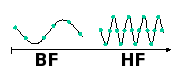###### In high frequencies the wavelength is small and the number of degrees of freedom is large

Due to limited capabilities of computers, finite element softwares cannot perform calculations up to the high frequency range.

 structure car aircraft ship frequency ~500 Hz ~50 Hz ~5 Hz
###### Maximal frequency by finite element method for a model of the order of million degrees of freedom.

The increase of frequency also induces an increase of the number of natural modes of structure. Rapidly, the population of modes becomes very large. Thus, the mathematical methods based on the development of solution on modal basis become intractable.

Numerical models require the knowledge of many parameters such as exact geometry, material properties, applied forces... But some of them are generally not well-known and we must consider that all input data have an uncertainty. However, results of computation become more sensitive to uncertainty when the frequency increases. So, even if we had very strong computers capable of doing calculations up to infinite frequency, those results would be not reliable.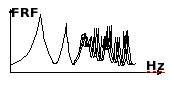### The principles of SEA.

SEA is a recent theory of vibroacoustics [1, 2]. This is a theory well suited to complex structures vibrating in the high frequency domain. It is based on the application of concepts and methods of statistical physics to the study of energy exchange between acoustical and mechanical components. The pioners of SEA were largely inspired by statistical physics and recent advances in SEA continue to be inspired by it. SEA is a demonstration that in engineering sciences, conceptual revolution is still possible.

Modes are the sites where energy is localized. They play a crucial role in SEA. Their number is assumed to be large (large population) and no mode plays a particular role (no dominant individual behaviour). Vibrating and acoustical sources are random. Then, the repartition of vibrational energy over modes is in perpetual modification.

Modes are grouped to constitute sub-systems. A sub-system is defined as a packet of similar modes in which energy is equally shared. They can be structural components like rod, beam, plate, shell, acoustical cavity but, they can be also a mode packet within a structural component like flexural modes in plate or longitudinal modes or torsional modes in rods. The most important assumption defining sub-systems is the equipartition of energy. Whithin all sub-systems, vibrational energy is shared between modes. Equipartition means that the time-averaged energy is the same for all modes of a sub-system.

A structure is thus divided in n sub-systems. This decomposition is macroscopic in the sense that all sub-systems must contain much modes. Sub-systems must therefore not be too small. Typically, a complex structure can be divided in a couple of dozen or hundreds sub-systems at most.###### Complex structures are splitted in structural or acoustical components called sub-systems.

Sub-systems behave like tanks containing various forms of energy, acoustical or vibrational, longitudinal, transverse, flexural waves... These tanks can receive, can dissipate but also can exchange energy with their neighbours. The common features to these different kinds of energy are that they are all localized in the same frequency bandwidth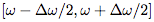and that they can be converted each other. SEA is the theory which describes these exchanges of energy. SEA is like thermodynamics of acoustical and structural vibrations, confined within the audio frequency band, 20 Hz et 20 kHz, which is very low compared with thermal frequencies of usual thermodynamics.### Energy and SEA.

All sub-systems can receive vibrational energy supplied by random sources, can dissipate energy and can exchange energy with neighbour sub-systems. In steady-state regime, the energy balance of sub-system i reads,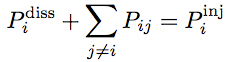(1)
where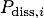is the dissipated power,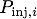is the power being injected by sources and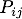is the net power exchanged with sub-system j.

The power dissipated in sub-system i is proportional to its vibrational energy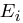. One introduces the so-called damping loss factor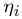such that,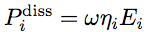(2)
where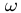is the central circular frequency (rad/s) of the octave band.

Other energy exchanges are taken into account in a similar manner than internal dissipation. Thus, the energy lost by sub-system i towards sub-system j reads,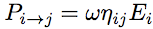where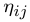is called coupling loss factor. This equation is similar to previous one for internal dissipation. It must also be considered that sub-system j can provide sub-system i with,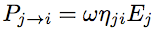The net exchanged power is the difference of both contributions,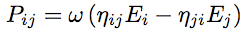(3)

Coupling loss factorsand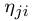are not independent. They are linked by the reciprocity relationship,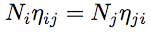(4)
where Ni is the number of modes of sub-system i. The exchanged power can then be re-written as the difference of modal energies.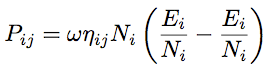(5)

By substituting equations (2) and (5) into the power balance (1), one obtains a set of linear equations on the unknowns. However, this system is not symmetric. The usage in SEA is not to write it on total energiesbut rather on modal energies Ei/Ni. We get,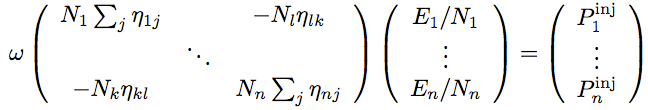(6)
This is a symmetric system that can be solved by any standard method. All these considerations on energy in SEA can be summarized in the following figure,### Entropy and SEA.

A SEA sub-system is a packet of N resonators whith increasing natural frequencies. Its vibrational energy is E. But since sources are random, the repartition of this energy over the N modes is fluctuating. The only mean energy E is supposed to be known.The entropy associated to this dynamical system is a measure of the number of possibilities to share energy E over N modes. This is ,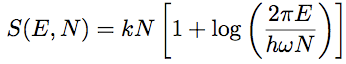(6)
where k is Boltzmann constant and h is Planck constant. This entropy is a state function which only depends on the extensive quantities vibrational energy E number of modes N. The vibrational temperature is defined as for any thermodynamical system by,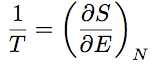One obtains,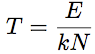(7)
The vibrational temperature is therefore equal to the modal energy divided by Boltzmann constant. The number of modes of a sub-system is constant. Only the vibrational energy can vary. The variation of entropy is therefore obtained by differentiating (6) thus, dS=dE/T. In case of a single source of power P, the energy supplied to sub-system during dt is dE=Pdt. The vibrational temperature being T=E/kN, the rate of entropy created by the source is,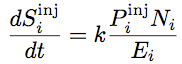This rate is positive since the source supplies energy to sub-system and therefore warms it. In a similar way, the rate of entropy due to dissipation can be calculated. It yields,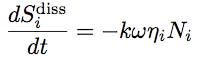We can observe that this rate is negative. Dissipation processes therefore tend to decrease the entropy level. This is the converse of what happens in thermodynamics! This result can be explained by the fact that here, dissipation is responsible of a decrease of internal energy and therefore cool down the system. Indeed, this decrease must be accompanied by an increase of the thermodynamical entropy. Finally, one can calculate the entropy rate created during the energy exchange between two adjacent sub-systems. It yields,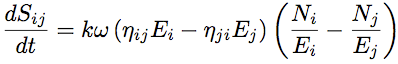It is always positive as it can be easily checked. This is the expression of the second principle of thermodynamics in the particular framework of SEA. The global entropy balance is the following,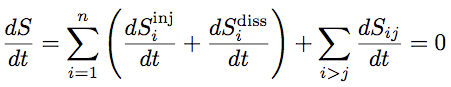which is summarized in the following diagram,### Validity of SEA.

Four parameters are required to define the validity domain of SEA [3, 5]

• The number of modes N in the octave band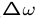must be large,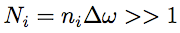where niThe modal overlap M is,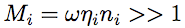A large modal overlap means that modes are not distinguishable. It ensures that fluctuations of energy about the mean value predicted by SEA are low.
• The coupling factor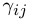between connected sub-systems,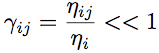A light coupling factor is necessary to authorize a local exchange of energy while maintaining a diffuse field (thermal equilibrium) in sub-systems.
• The normalized attenuation factor m,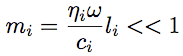This factor ensures that thermal equilibrium (diffuse field) holds within sub-systems without being disturbed by a too strong internal dissipation.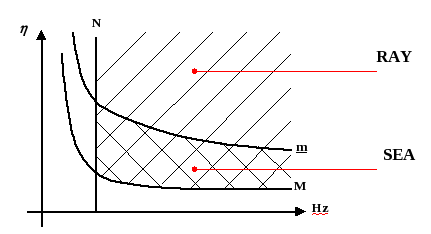###### Validity domain of SEA for flexural vibration of a single plate.

In the frequency - damping space, these four parameters limit the validity domain of SEA. The line N=cte is vertical, the lines m=cte and M=cte are hyperbolas. The validity domain of SEA is a strip extending up to high frequencies. The ray method only requires the two conditions N>>1 and M>>1 without the two other conditions so that the validity domain of rays is larger than those of SEA.

### A recent theory.

1958, Lyon, Eishler, Maidanik... laid down the basic ideas by the modal approach.
1975, Publication of the first book on SEA by R.H. Lyon 
1980, Wave approach of SEA
1990, Diffusion of SEA in all industries
1995, Thermal generalizations of SEA

### Some applications.

All fields of mechanical industry are concerned, aerospace, automotive, railway, energy, weapon... SEA is a powerful tool for design and optimization of structures. It allows to know energy fluxes and therefore to elaborate efficient solutions to stop the flowing of vibrational energy.

Nowadays, SEA has been adopted by almost all industries. SEA has been attractive to designers mainly because of its original insight into structural dynamics more than the efficiency of its predictive results.

### Towards non-equilibrium thermodynamics…

The main difficulty encountered when applying SEA is the generally too low number of degrees of freedom in usual systems of order of few hundreds modes to be compared with Avogadro's number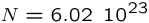in statistical physics. This has two consequences: Fluctuations about mean behaviour are very important and vibrational fields are often not diffuse. These two shortcomings motivate an active research in laboratories up to day. The question of uncertainty in SEA is strongly connected to fluctuation theorem in statistical physics. Those of the breaking of diffuse field assumption requires the elaboration of a theory of statistical vibroacoustics beyond equilibrium analogous to the kinetic theory of gases in thermodynamics. This is what we have done with the radiative transfer equation.

### Bibliography.

 R.H. Lyon, Statistical Energy Analysis of Dynamical Systems: Theory and Application, Cambridge, Massachusetts, MIT Press, 1975.

 R.H. Lyon and R.G. DeJong, Theory and Application of Statistical Energy Analysis, Boston, Butterworth-Heinemann, 1995.

 A. Le Bot, Derivation of statistical energy analysis from radiative exchanges, Journal of Sound and Vibration, vol. 300, p. 763-779, 2007.

 A. Le Bot, Entropy in statistical energy analysis, Journal of the Acoustical Society of America, vol. 125, p. 1473-1478 , 2009.

 A. Le Bot and V. Cotoni, Validity diagrams of statistical energy analysis, Journal of Sound and Vibration, vol. 329, p. 221-235, 2010.

 A. Le Bot, A. Carcaterra, D. Mazuyer, Statistical vibroacoustics and entropy concept, Entropy, vol. 12, p. 2418-2435, 2010.